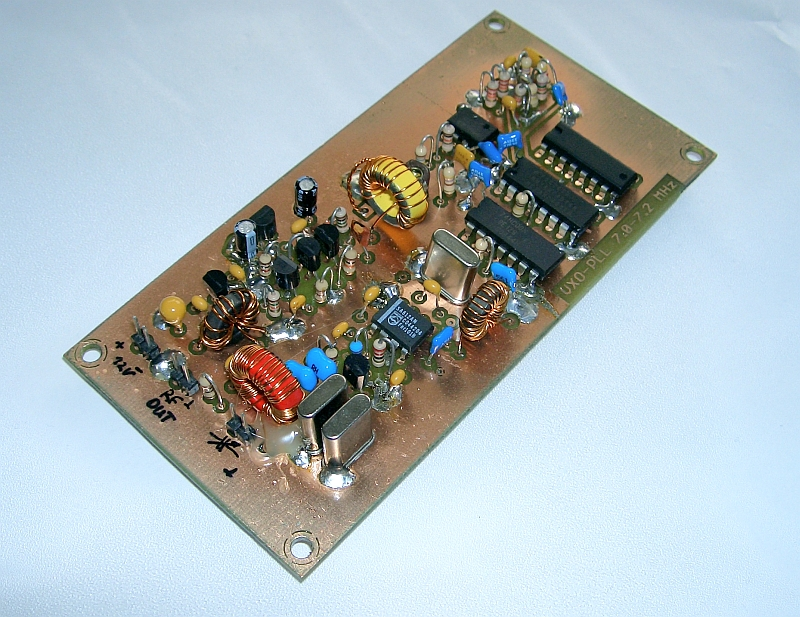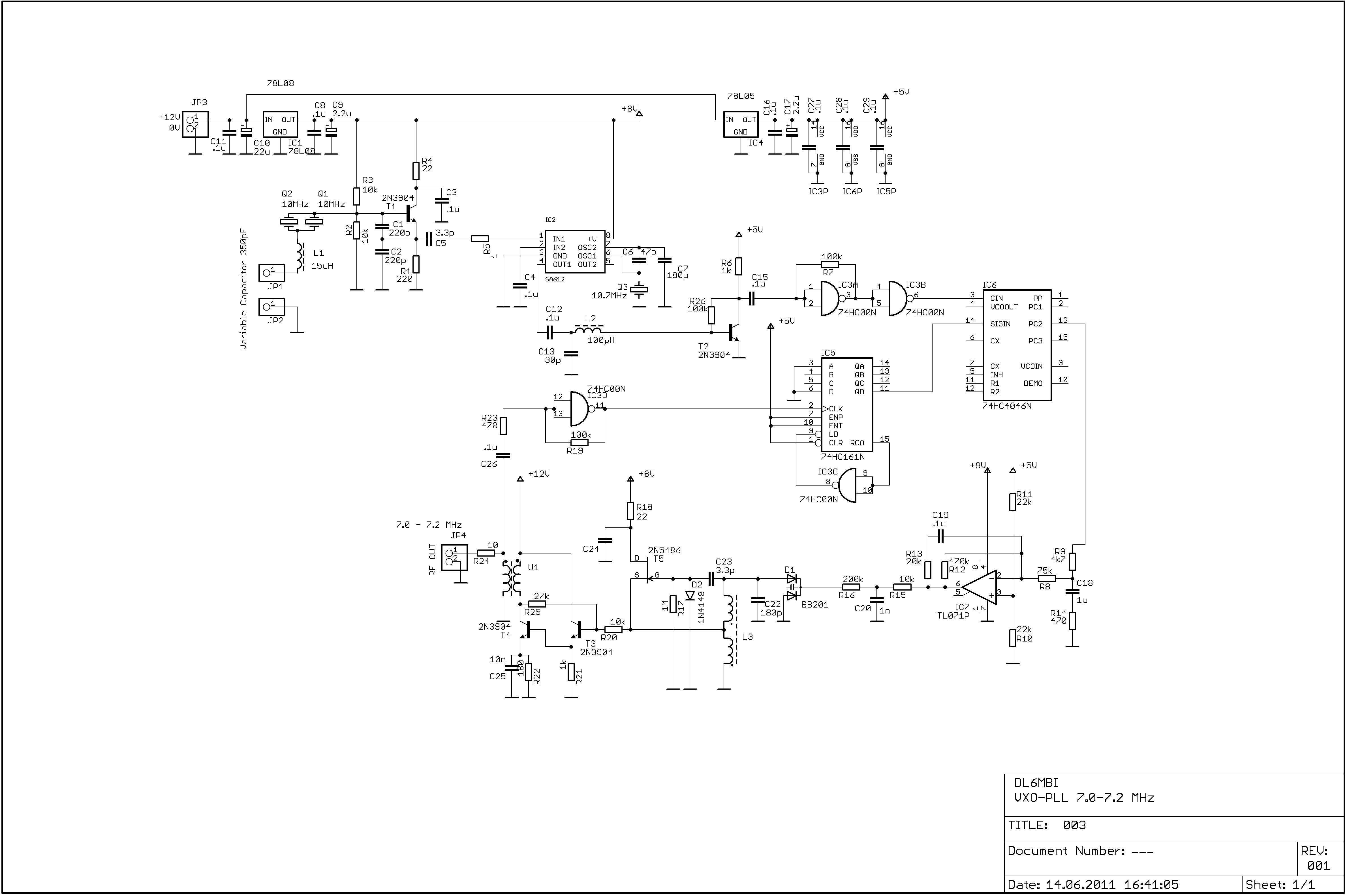Flexible VXO-PLL Oscillator
DL6ZB, Rolf Heine (ex DL6MBI)

PLL/DDS frequency generation systems are complex and need sophisticated programming and measurement. On the other hand a lot of home-brewers don't want to use microprocessor driven PLL circuits or DDS systems, although they don't want to miss rock stable frequency generation. Here is a simple, low-cost but very effective solution for this.Picture: Example of a 7.0-7.2 MHz VXO-PLL. Variable capacitor (approx. 350pF) isn't shown. It is connected to the front side connector (see capacitor symbol).

This VXO-PLL actually works on 40m for direct conversion receivers and simple transmitters, although it is usable for other frequencies and IF systems. The output frequency depends on the divider ratio (74HC161) and the VCO frequency range. I use this VXO-PLL for my direct conversion QRP transceiver Type 002 project. The tuning element is a simple variable capacitor. A varicap diode is also suitable as a tuning element for this.

How does ist work?

Example:
• VCO frequency range = 7.0-7.2 MHz
• 74HC161 dividing factor = 10
• VXO crystal frequency = 10.000 MHz
• CO crystal frequency = 10.700 MHz
Theory of operation:

The VXO output is mixed with the CO frequency (10.7 MHz) of the SA612s internal crystal oscillator. The SA612 output frequency (f1-f2) is approximately 700 kHz (+/-).

Now suppose the VXO will be tuned down a few kHz (to about 25 kHz max.), then the SA612s output frequency rises because the difference between the CO frequency and the VXO-frequency spreads.

Example: CO frequency = 10.7 MHz. VXO frequency = 9.985 MHz
f(ref) is then:  10.700 - 9.985 = 715 kHz. The VCO output frequency is multiplied by the 74161 division factor=10. The output frequency now is tuned to 7.150 MHz.

Why 7.150 MHz ?

The VCO output frequency is divided by 10 by the 74HC161 divider. The divided VCO output frequency at the 74HC161 divider is now in the tuning range of 700-725 kHz. The 74HC161 divider output will now be phase/frequency compared with the SA612s output (after signal forming) by the 74HC4046 phase/frequency comparator. The output of the 74HC4046 is low-pass filtered through the TL071 VCO loop filter. The loop filter DC output drives the BB201 varactor diode. This VCO design was choosen because it is broadband, low noise, simple, low cost and reliable.

This VXO-PLL principle can be used for any frequency by adjusting the divider and/or crystal frequencies. It is very flexible. Use any divider with division ratios higher than 16 for 20-10m output.

Divider Factors vs. Output Frequency:

 Factor Frequeny/MHz 5 3.5-3.6 10 7.0-7.2 20 14.0-14.4 30 21.0-21.6 40 28.0-28.8